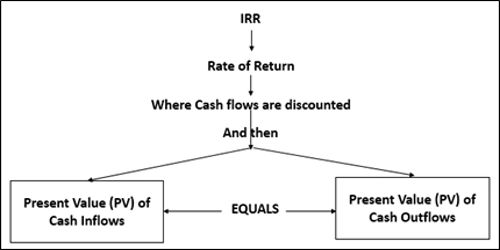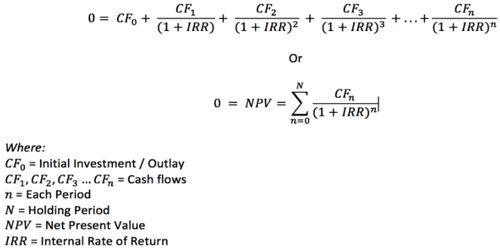# Internal Rate of Return (IRR)

The internal rate of return, or IRR, is one of the most popular methods of evaluating potential projects. The discount rate often used in capital budgeting that makes the net present value of all cash flows from a particular project equal to zero. It is the interest rate at which the net present value of all the cash flows (both positive and negative) from a project or investment equal zero. It is a metric used in capital budgeting to estimate the profitability of potential investments. The internal rate of return is typically used to calculate the profitability of investments made in a financial product or projects

IRR Formula –Generally speaking, the higher a project’s internal rate of return, the more desirable it is to undertake the project. As such, IRR can be used to rank several prospective projects a firm is considering. Assuming all other factors are equal among the various projects, the project with the highest IRR should probably be considered the best and undertaken first.